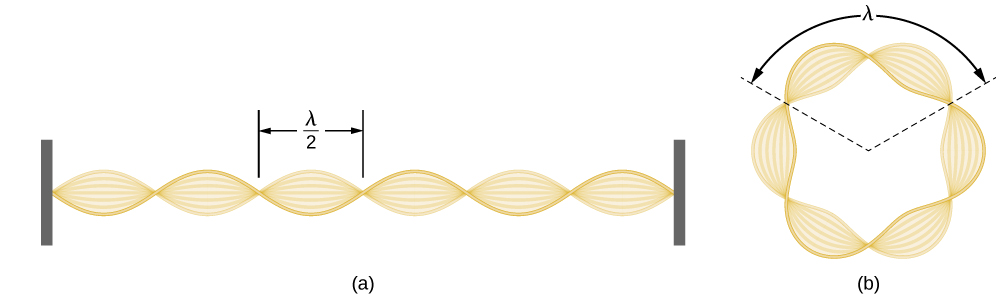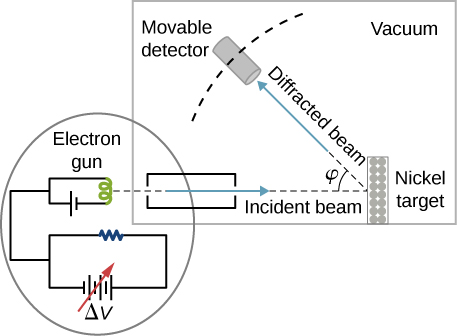# 6.5 De broglie’s matter waves  (Page 3/10)

 Page 3 / 10Standing-wave pattern: (a) a stretched string clamped at the walls; (b) an electron wave trapped in the third Bohr orbit in the hydrogen atom.

## The electron wave in the ground state of hydrogen

Find the de Broglie wavelength of an electron in the ground state of hydrogen.

## Strategy

We combine the first quantization condition in [link] with [link] and use [link] for the first Bohr radius with $n=1.$

## Solution

When $n=1$ and ${r}_{n}={a}_{0}=0.529\phantom{\rule{0.2em}{0ex}}\text{Å,}$ the Bohr quantization condition gives ${a}_{0}p=1·\hslash ⇒p=\hslash \phantom{\rule{0.1em}{0ex}}\text{/}\phantom{\rule{0.1em}{0ex}}{a}_{0}.$ The electron wavelength is:

$\lambda =h\phantom{\rule{0.1em}{0ex}}\text{/}\phantom{\rule{0.1em}{0ex}}p=h\phantom{\rule{0.1em}{0ex}}\text{/}\phantom{\rule{0.1em}{0ex}}\hslash \phantom{\rule{0.1em}{0ex}}\text{/}\phantom{\rule{0.1em}{0ex}}{a}_{0}=2\pi {a}_{0}=2\pi \left(0.529\phantom{\rule{0.2em}{0ex}}\text{Å}\right)=3.324\phantom{\rule{0.2em}{0ex}}\text{Å}.$

## Significance

We obtain the same result when we use [link] directly.

Check Your Understanding Find the de Broglie wavelength of an electron in the third excited state of hydrogen.

$\lambda =2\pi n{a}_{0}=2\text{(}3.324\phantom{\rule{0.2em}{0ex}}\text{Å}\text{)}=6.648\phantom{\rule{0.2em}{0ex}}\text{Å}$

Experimental confirmation of matter waves came in 1927 when C. Davisson and L. Germer performed a series of electron-scattering experiments that clearly showed that electrons do behave like waves. Davisson and Germer did not set up their experiment to confirm de Broglie’s hypothesis: The confirmation came as a byproduct of their routine experimental studies of metal surfaces under electron bombardment.

In the particular experiment that provided the very first evidence of electron waves (known today as the Davisson–Germer experiment    ), they studied a surface of nickel. Their nickel sample was specially prepared in a high-temperature oven to change its usual polycrystalline structure to a form in which large single-crystal domains occupy the volume. [link] shows the experimental setup. Thermal electrons are released from a heated element (usually made of tungsten) in the electron gun and accelerated through a potential difference $\text{Δ}V,$ becoming a well-collimated beam of electrons produced by an electron gun. The kinetic energy K of the electrons is adjusted by selecting a value of the potential difference in the electron gun. This produces a beam of electrons with a set value of linear momentum, in accordance with the conservation of energy:

$e\text{Δ}V=K=\frac{{p}^{2}}{2m}⇒p=\sqrt{2me\text{Δ}V}.$

The electron beam is incident on the nickel sample in the direction normal to its surface. At the surface, it scatters in various directions. The intensity of the beam scattered in a selected direction $\phi$ is measured by a highly sensitive detector. The detector’s angular position with respect to the direction of the incident beam can be varied from $\phi =0\text{°}$ to $\phi =90\text{°}.$ The entire setup is enclosed in a vacuum chamber to prevent electron collisions with air molecules, as such thermal collisions would change the electrons’ kinetic energy and are not desirable.Schematics of the experimental setup of the Davisson–Germer diffraction experiment. A well-collimated beam of electrons is scattered off the nickel target. The kinetic energy of electrons in the incident beam is selected by adjusting a variable potential, Δ V , in the electron gun. Intensity of the scattered electron beam is measured for a range of scattering angles φ , whereas the distance between the detector and the target does not change.

When the nickel target has a polycrystalline form with many randomly oriented microscopic crystals, the incident electrons scatter off its surface in various random directions. As a result, the intensity of the scattered electron beam is much the same in any direction, resembling a diffuse reflection of light from a porous surface. However, when the nickel target has a regular crystalline structure, the intensity of the scattered electron beam shows a clear maximum at a specific angle and the results show a clear diffraction pattern (see [link] ). Similar diffraction patterns formed by X-rays scattered by various crystalline solids were studied in 1912 by father-and-son physicists William H. Bragg and William L. Bragg . The Bragg law in X-ray crystallography provides a connection between the wavelength $\lambda$ of the radiation incident on a crystalline lattice, the lattice spacing, and the position of the interference maximum in the diffracted radiation (see Diffraction ).

what is light
What is photoelectric
light energy (photons) through semiconduction of N-P junction into electrical via excitation of silicon purified and cristalized into wafers with partially contaminated silicon to allow this N-P function to operate.
Michael
i.e. Solar pannel.
Michael
If you lie on a beach looking at the water with your head tipped slightly sideways, your polarized sunglasses do not work very well.Why not?
it has everything to do with the angle the UV sunlight strikes your sunglasses.
Jallal
this is known as optical physics. it describes how visible light, ultraviolet light and infrared light interact when they come into contact with physical matter. usually the photons or light upon interaction result in either reflection refraction diffraction or interference of the light.
Jallal
I hope I'm clear if I'm not please tell me to clarify further or rephrase
Jallal
what is bohrs model for hydrogen atom
hi
Tr
Hello
Youte
Hi
Nwangwu-ike
hi
Siddiquee
hi
Omar
helo
Mcjoi
what is the value of speed of light
1.79×10_¹⁹ km per hour
Swagatika
what r dwarf planet
what is energy
কাজের একক কী
Jasim
কাজের একক কী
Jasim
friction ka direction Kaise pata karte hai
friction is always in the opposite of the direction of moving object
Punia
A twin paradox in the special theory of relativity arises due to.....? a) asymmetric of time only b) symmetric of time only c) only time
b) symmetric of time only
Swagatika
fundamental note of a vibrating string
every matter made up of particles and particles are also subdivided which are themselves subdivided and so on ,and the basic and smallest smallest smallest division is energy which vibrates to become particles and thats why particles have wave nature
Alvin
what are matter waves? Give some examples
according to de Broglie any matter particles by attaining the higher velocity as compared to light'ill show the wave nature and equation of wave will applicable on it but in practical life people see it is impossible however it is practicaly true and possible while looking at the earth matter at far
Manikant
a centeral part of theory of quantum mechanics example:just like a beam of light or a water wave
Swagatika
Mathematical expression of principle of relativity
given that the velocity v of wave depends on the tension f in the spring, it's length 'I' and it's mass 'm'. derive using dimension the equation of the wave
What is the importance of de-broglie's wavelength?
he related wave to matter
Zahid
at subatomic level wave and matter are associated. this refering to mass energy equivalence
Zahid
it is key of quantum
Manikant
how those weight effect a stable motion at equilibriumBy Sam LuongBy Melinda SalzerBy Prateek AshtikarBy Kimberly NicholsBy David CoreyBy Brooke DelaneyBy OpenStaxBy Brooke DelaneyBy OpenStaxBy Richley Crapo